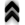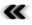### 4.1 Bare Basics

Silently assuming basic familiarity with the entity known as a set , several distinctions connected to sets should not go undeﬁned, especially as later concepts build on these very basic deﬁnitions and the lack of a clear distinction can be nothing but detrimental. Among others, Deﬁnition 36 is a higher level representative of the concept provided in the following deﬁnition.

Deﬁnition 1 (Open cover) An open cover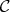of a set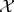is a family of open sets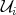, whose union results in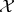: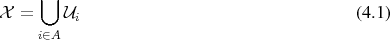with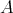being an arbitrary index set.

A similar, yet distinct concept is given in the next deﬁnition.

Deﬁnition 2 (Partition of a set) A partition of a set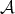is a set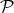of non-empty subsets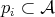, which fulﬁl the following conditions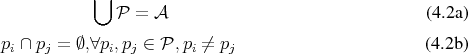Thus a partition implies that every element of the set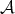is assigned to exactly one element of the partition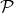.

To extend the simple notion of sets and their partitions, the following deﬁnition is of high utility.

Deﬁnition 3 (Cartesian Product) The Cartesian product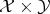of two sets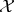and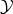is deﬁned as the set of all ordered tuples of the form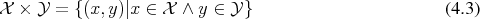It is through this simple mechanism represented by the Cartesian product that extensions to higher dimensions are available not only in a theoretical, but especially in an applied setting.

Sets alone present elements and very basic predicates such as testing, if a given element is contained within a set. It is, however, important to provide a more involved mechanism which is readily found in relations 1 .

Deﬁnition 4 (Relation) Considering two sets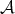and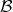, a relation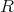is deﬁned as a subset of the Cartesian product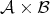.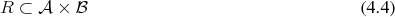A relation is not only an important step for further theoretical reﬁnement, but has also been a driving abstraction for digital data storage systems such as databases.

Relations can be further qualiﬁed with attributes in case they conform to certain requirements. A selection of qualiﬁers is provided in order to keep subsequent deﬁnitions from becoming unnecessarily convoluted.

Deﬁnition 5 (Reﬂexive relation) A binary relation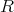on a set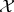is called reﬂexive, if every element is related to itself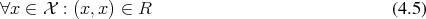Deﬁnition 6 (Symmetric relation) A binary relation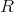on a set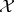is called symmetric, if the following is always true: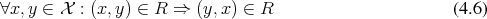Deﬁnition 7 (Transitive relation) A binary relation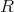on a set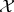is called transitive, if it follows that: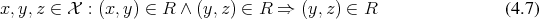Deﬁnition 8 (Equivalence relation) A binary relation which is reﬂexive, transitive and symmetric is called an equivalence relation.

The application of an equivalence relation to the elements of a set leads to the concept of equivalence classes, as deﬁned in the following.

Deﬁnition 9 (Equivalence class) Given an equivalence relation, equivalence classes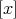are deﬁned as the sets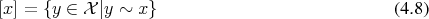Equivalence classes are of special interest as they provide a means to partition a set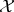. The demands placed on equivalence relations (Deﬁnition 8) ensure that a set of equivalence classes (Deﬁnition 9) is indeed a partition of the set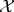(Deﬁnition 2):

• Reﬂexivity (Deﬁnition 5) ensures that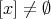• Symmetry (Deﬁnition 6) ensures• Transitivity (Deﬁnition 7) asserts that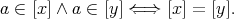In other words, two elements are either in the same equivalence class, or in disjoint classes. An element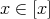is called a representative of the equivalence class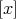.

The memory within digital computers is conceptually a set, because every cell of memory can be distinctly addressed even without having to resort to querying the contents of the cells, thus it is really the structure of the cells that forms a set. The presented relations are only a small fraction of the relations available and indeed required to represent modern memory management, but are at least suﬃcient to cover theoretical considerations.